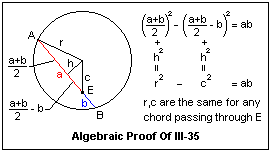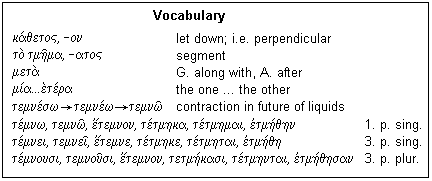## Lesson κγ': Chords in a Circle

This remarkable proposition shows the power of combining geometry and algebra

Book III, Proposition 35 states: When two chords in a circle cut one another, the rectangle enclosed by the segments of one is equal to the rectangle enclosed by the segments of the other. This is surely not an obvious proposition, and its proof is essentially algebraic. It rests on Book II, Proposition 5, and shows how algebra is done in Euclid. The result is of frequent practical application, and is a valuable one.A partly algebraic proof is shown in the Figure. The chord AB is divided at point E into two segments. The product ab of the lengths of the segments is equal to the difference of the squares as shown, by the familiar rule for factoring a difference of squares. This is the result of Book II, Proposition 5. The distance from the center of the circle to the chord is h. This line, as we know, is normal to the chord and bisects it (Book III, Proposition 3). By adding h2 to each square, from Book I, Proposition 47 we get r2 and c2, respectively, which are the same for any chord passing through E, since r is the radius of the circle and c is the distance of E from the center. The result then follows immediately. A direct algebraic approach to the problem would be more difficult. The combination of geometry and algebra, however, finds the solution neatly and quickly, and provides insight and a reason for the result, not simply the bare result.The proposition as it appears in Euclid is shown at the right. There are few new words, if any, and the vocabulary below explains some of the principal words used here. The definite article is extensively used to help clarify and shorten an involved demonstration, so pay special care to the cases. The statement is: "If in a circle two straight lines cut one another, the rectangle enclosed by the segments of the one is equal to the rectangle enclosed by the segments of the other." Note the subjunctive after E)a/n, seen in the ω instead of ο. In terms of the figure, "Indeed, in the circle ABΓΔ let the straight lines AΓ, BΔ cut one another at the point E; I say, that the rectangle enclosed by AE, EΓ is equal to the rectangle enclosed by ΔE, EB."

Now the trivial case of the lines intersecting at the center of the circle is disposed of, since the method of proof to be used in general fails in this particular case, as we will see from the construction. "If indeed AΓ, BΔ are through the center, so that E is to be the center of circle ABΓΔ, And so [it is] clear that, AE, EΓ, ΔE, EB being equal, the rectangle enclosed by AE, EΓ is equal to the rectangle enclosed by ΔE, EB." I translate as "enclosed by" the word that literally means "around-having," perieco/menoj, a present middle participle.

First, the construction: "Let AΓ, ΔB not be through the center, and let the center of the circle be taken, and let it be Z, and from Z to AΓ, ΔB draw perpendicularly straight lines ZH, ZΘ and join ZB, ZΓ, ZE. Now, on to the demonstration. As explained above, the method of proof is to show that the area of the rectangle formed by the segments is the difference of squares that is the same for any chord. "And since a certain straight line HZ through the center cuts at right angles a certain straight line not through the center AΓ, it bisects this line. AH is, therefore, equal to HΓ. Therefore, since the straight line AΓ is cut into equals by H, and into unequals by E, therefore the rectangle bounded by AE, EΓ with the square on EH is equal to the [square on] HΓ Let the [common] square on HZ. Therefore, the [rectangle] on AE, EΓ with the [squares] on HE, HZ is equal to the [squares] on ΓH, HZ. However, the [squares] on EH, HZ are equal to the [square] on ZΓ. And ZΓ is equal to ZB. Therefore, the [rectangle] AE, EΓ with the [square] on EZ is equal to the [square] on ZB. In the same way, also the [rectangle] ΔE, EB with the [square] on ZE is equal to the [square] on ZB. It has been shown that the [rectangle] AE, EΓ with the [square] ZE is equal to the [square] on ZB. Therefore, the [rectangle] AE, EΓ with the [square] ZE is equal to the [rectangle] ΔE, EB with the [square] ZE. Take away the common [square] ZE; the remainder, therefore, the rectangle enclosed by AE, EΓ is equal to the rectangle enclosed by ΔE, EB."

Now, at last, comes the summing-up: "If, therefore, in a circle two straight lines cut one another, the rectangle enclosed by the segments of one is equal to the rectangle enclosed by the segments of the other; which was to be shown." A special case has not been considered, which arises when one line does not pass through the center, but the second one does. In this case, the product of the segments of the line that does pass through the center is easily shown to be the same difference of squares, since the center divides this line equally, and E divides it unequally. The result then follows immediately from Book II, Proposition 5.The word for perpendicularly, which is the neuter accusative singular of the adjective, comes from kata\-i/)hmi, to "let down", as a fishing line, which is perpendicular to the water. The preposition me/ta has different meanings when used with the genitive or accusative. Here, we see it used with the genitive, where it means "with" but it is most frequently seen with the accusative, where it means "after" in space or time. mi/a ... e/(tera is, of course, the feminine, which we see in the genitive, meaning "of the one [line]", "of the other [line]." It agrees with line, which is feminine, so it clearly shows we are dealing with the line, not the segments, and are saying "the segments of the line." The word for segment is used a lot here, and it comes from the verb te/mnw, "cut." This verb is one of a large class whose stems end in a liquid consonant, λ, μ, ν or ρ. The sound of such a consonant can be prolonged, unlike that of most consonants. The present tense comes from temne/w, but the ω absorbs the ε without a trace. In the future, this ε reappears, as shown, after a liquid, and the accent is even attracted to it. A σ between two vowels, as here, tends to disappear. Finally, the vowels contract, and the result looks like the present, but with a different accent. This happens also in verbs ending in -ιζω, and a few others as well. It is well to be aware of changes like this, which can confound the unwary. The six principal parts of the verb are also given, but in the third person as well, which we meet much more frequently.